# RS Aggarwal Solutions for Class 7 Maths Chapter 10 Percentage

Maths is a subject which requires understanding and reasoning skills with logic. Along with this, it also requires students to practice maths regularly. Students of Class 7 are suggested to solve RS Aggarwal Class 7 Solutions to strengthen the fundamentals and be able to solve questions that are usually asked in the examination. From all the chapters, Percentage is an important topic for the students, and one needs to practice thoroughly to score well in the exam. RS Aggarwal Solutions for Class 7 Maths Chapter 10 – Percentage are available here.

The main aim is to help students understand and crack these problems. We at BYJU’S have prepared the RS Aggarwal Solutions for Class 7 Maths Chapter 10 wherein problems are solved step by step with detailed explanations. Download pdf of Class 7 Chapter 10 in their respective links.

## Download PDF of RS Aggarwal Solutions for Class 7 Maths Chapter 10 – Percentage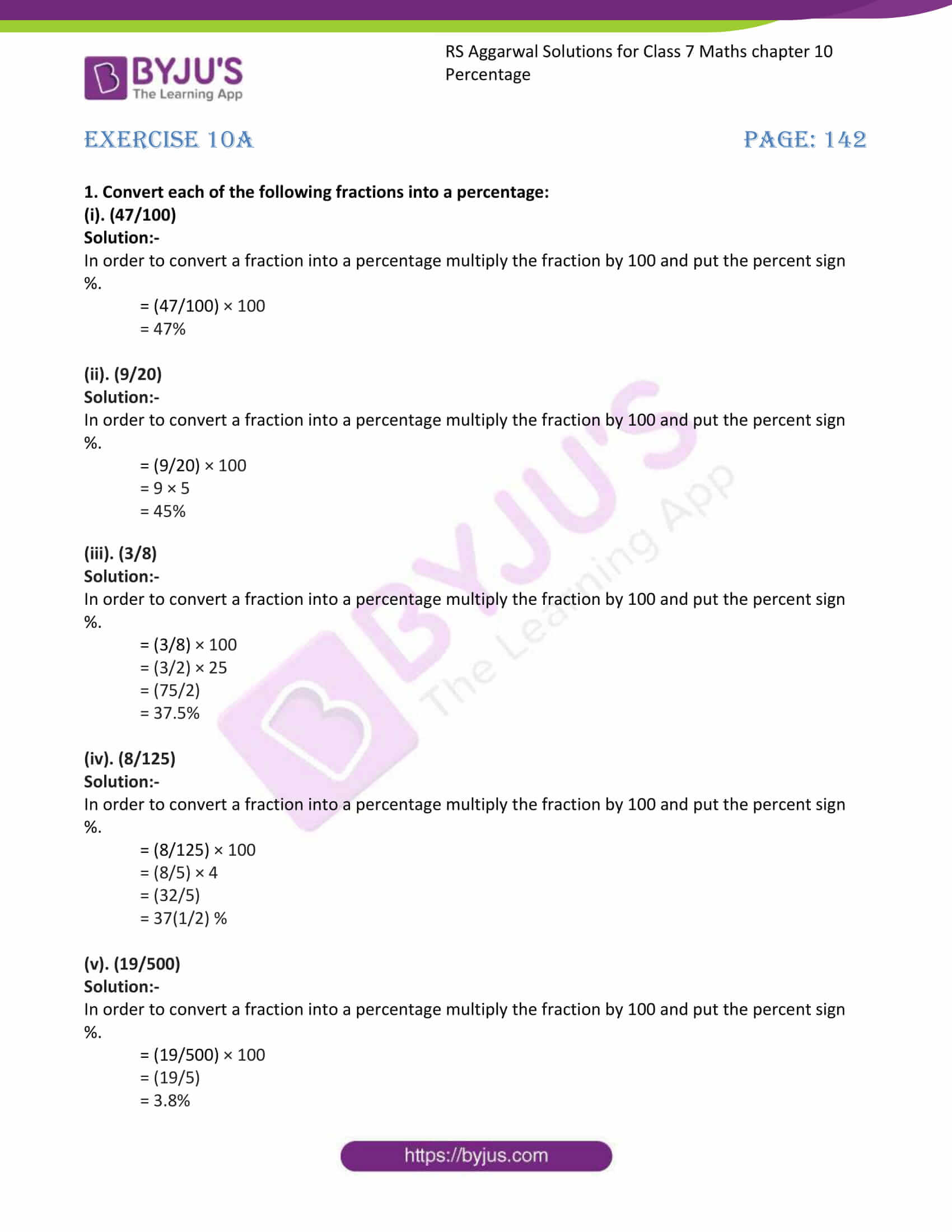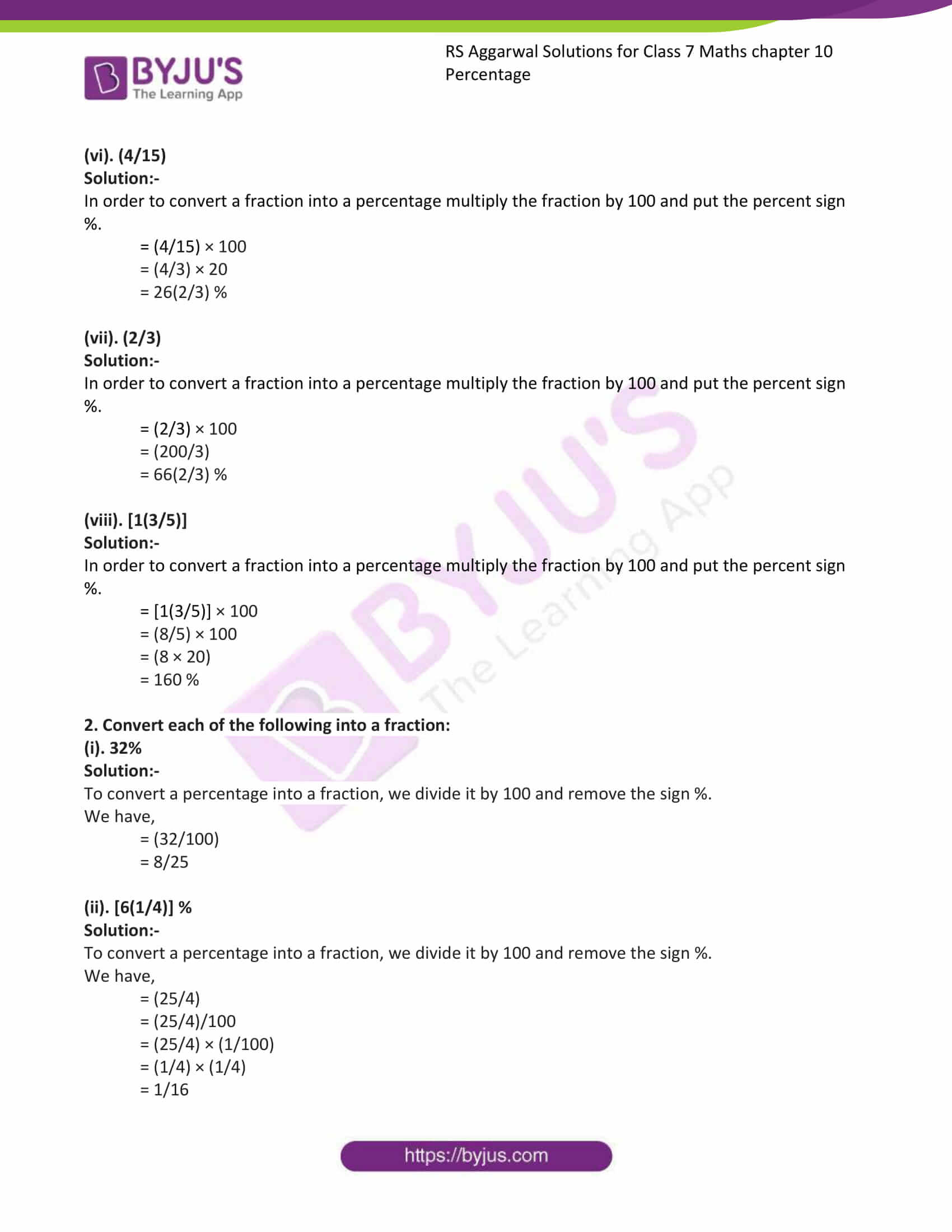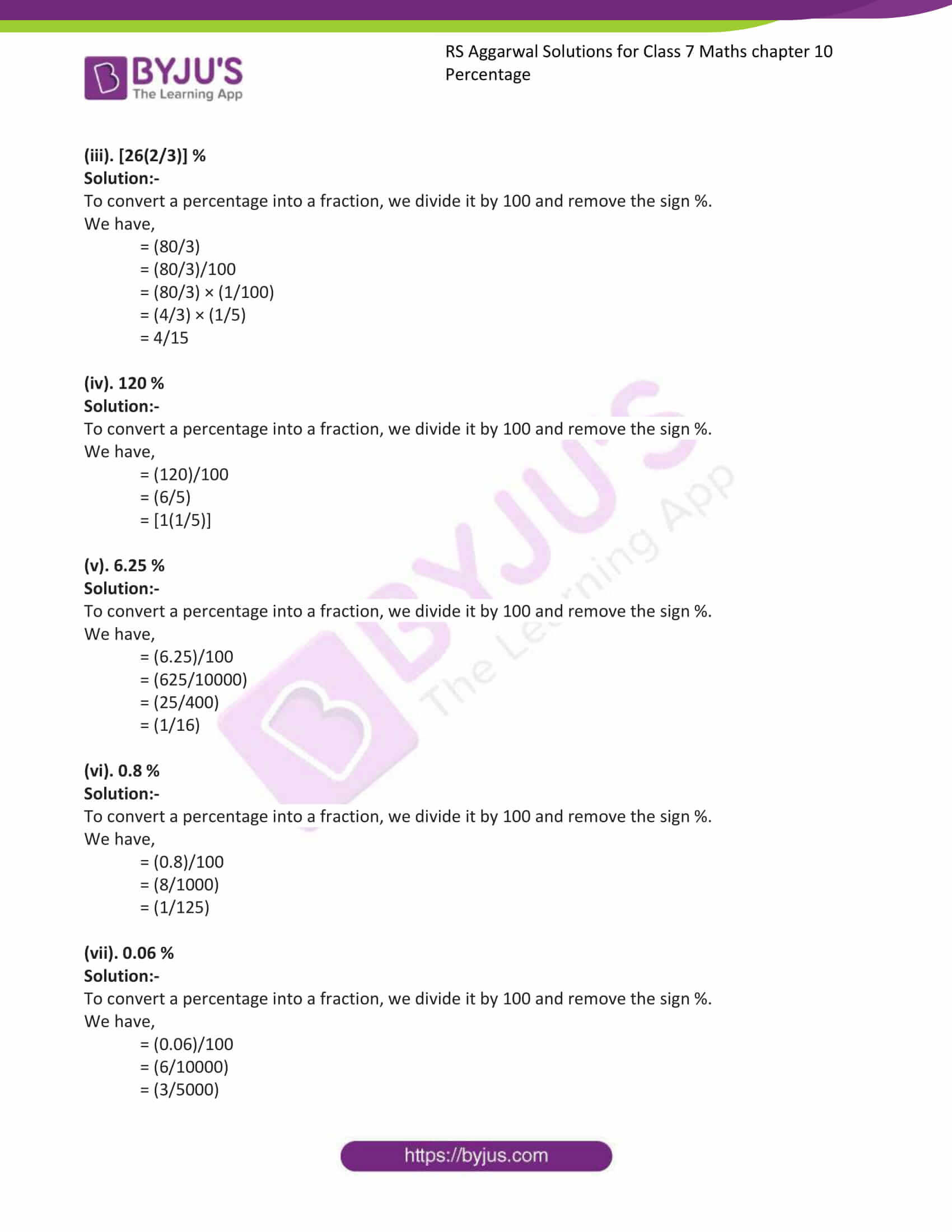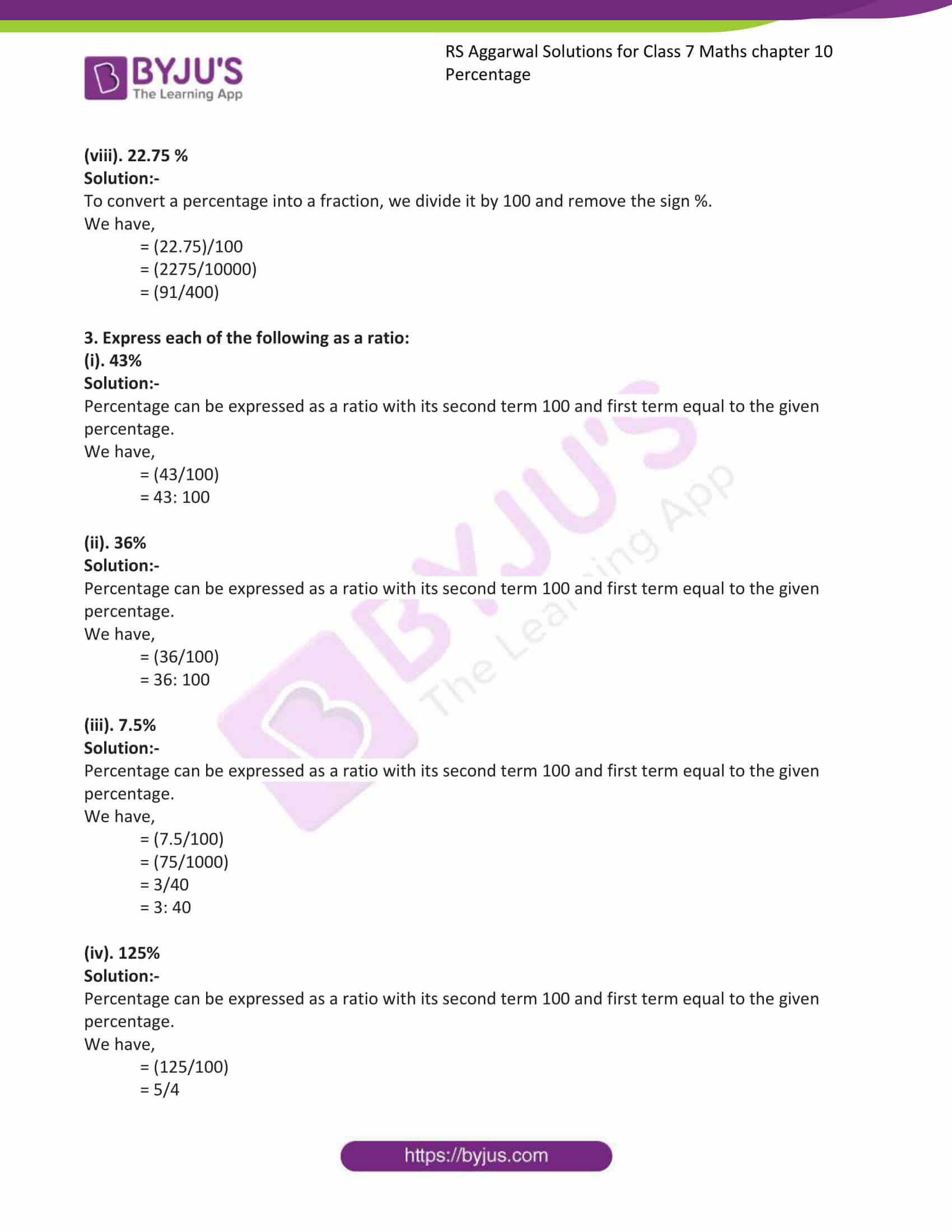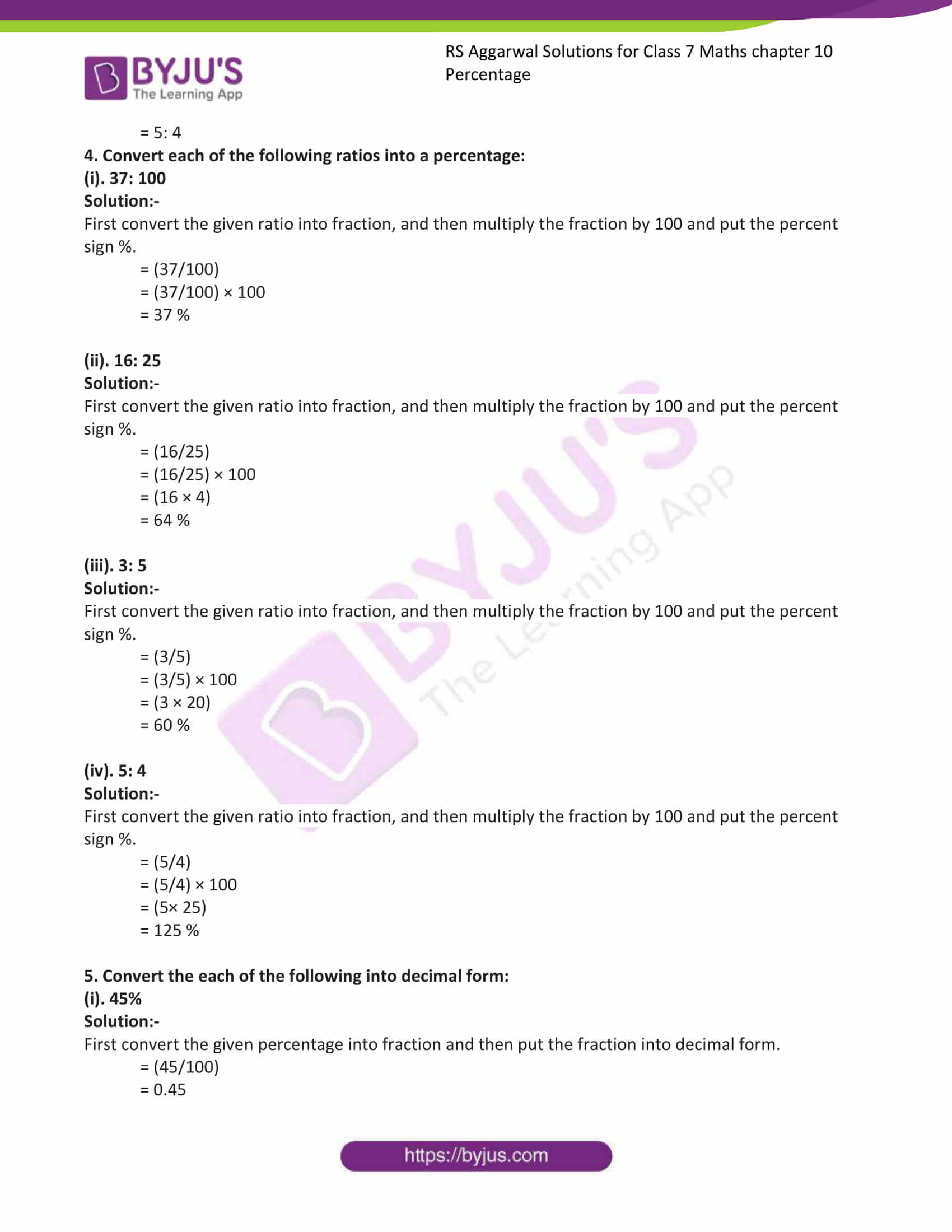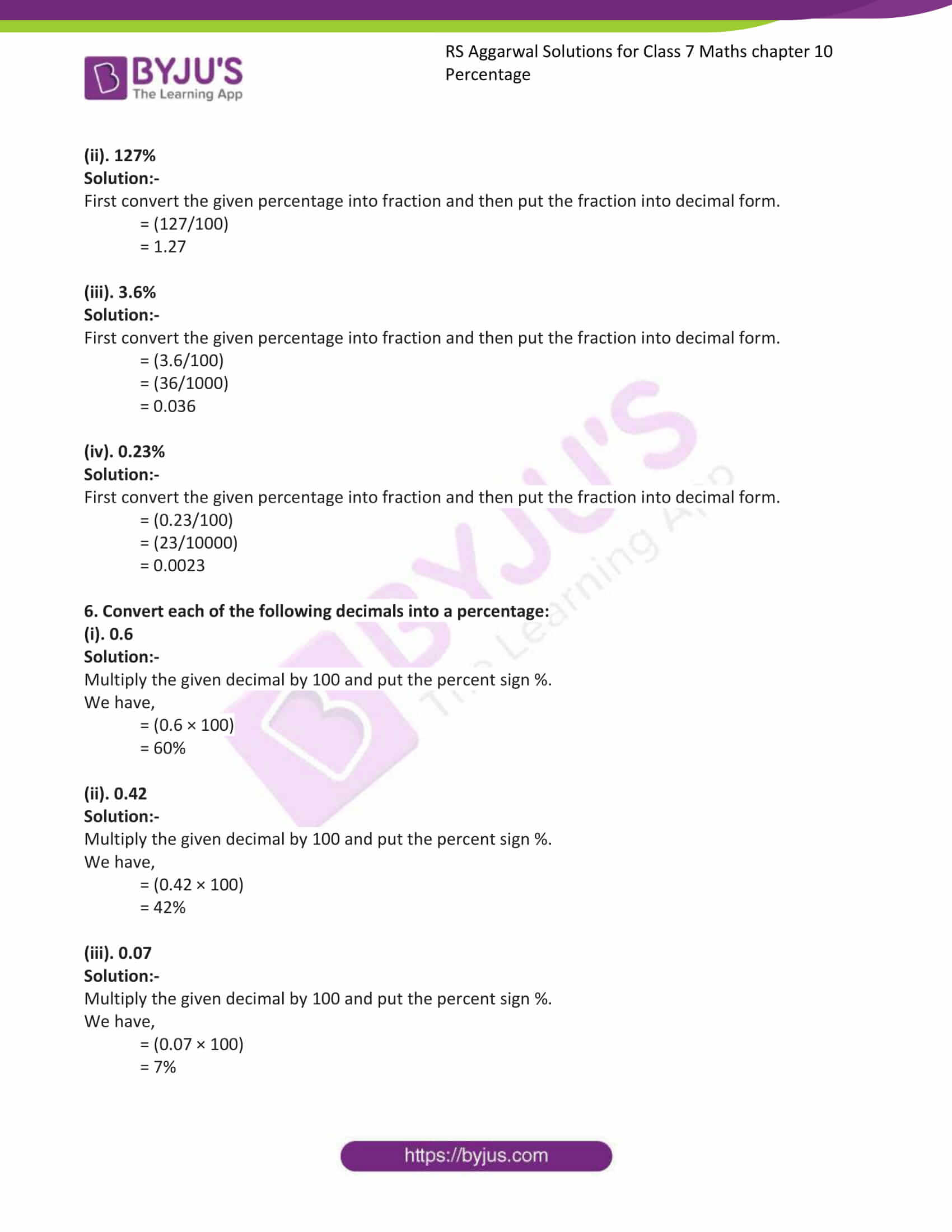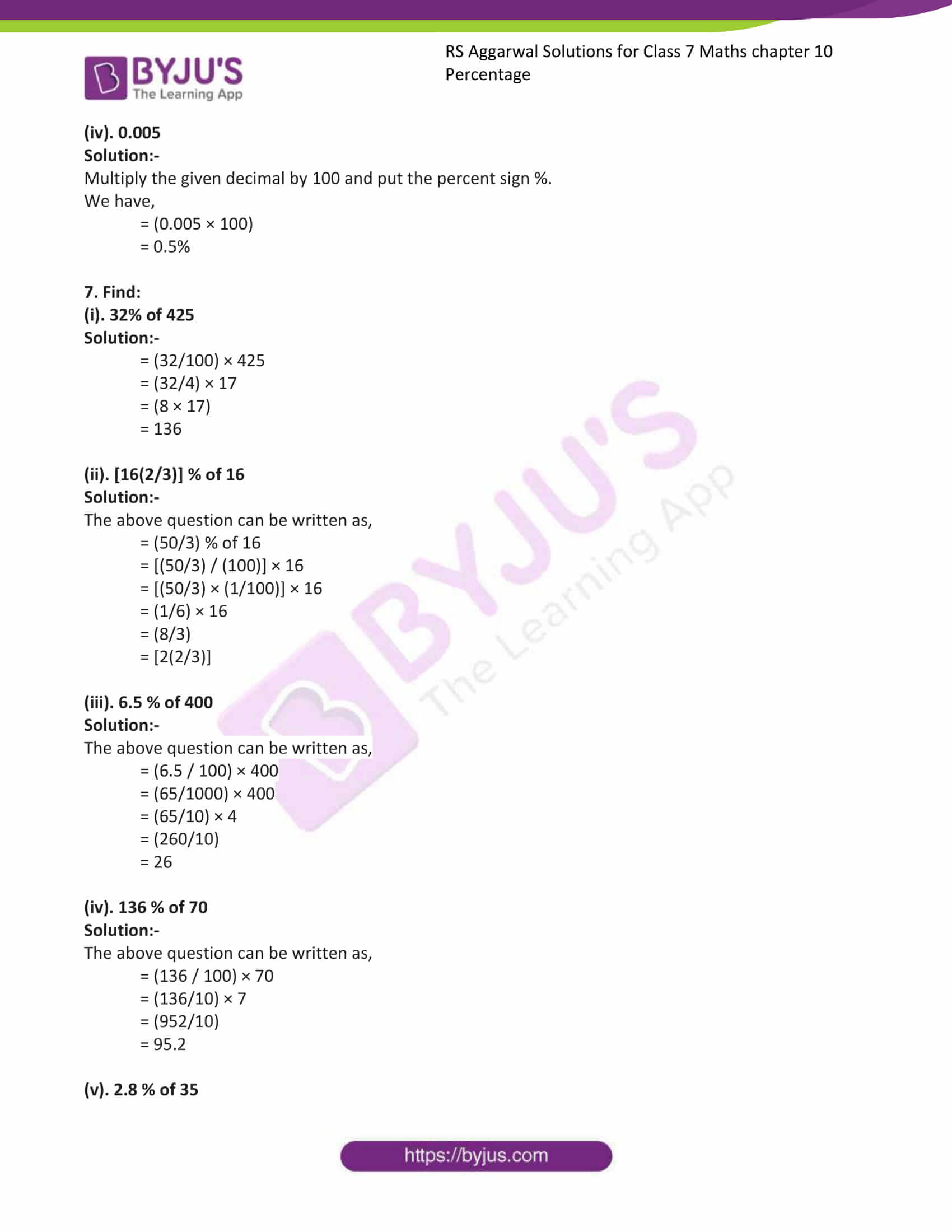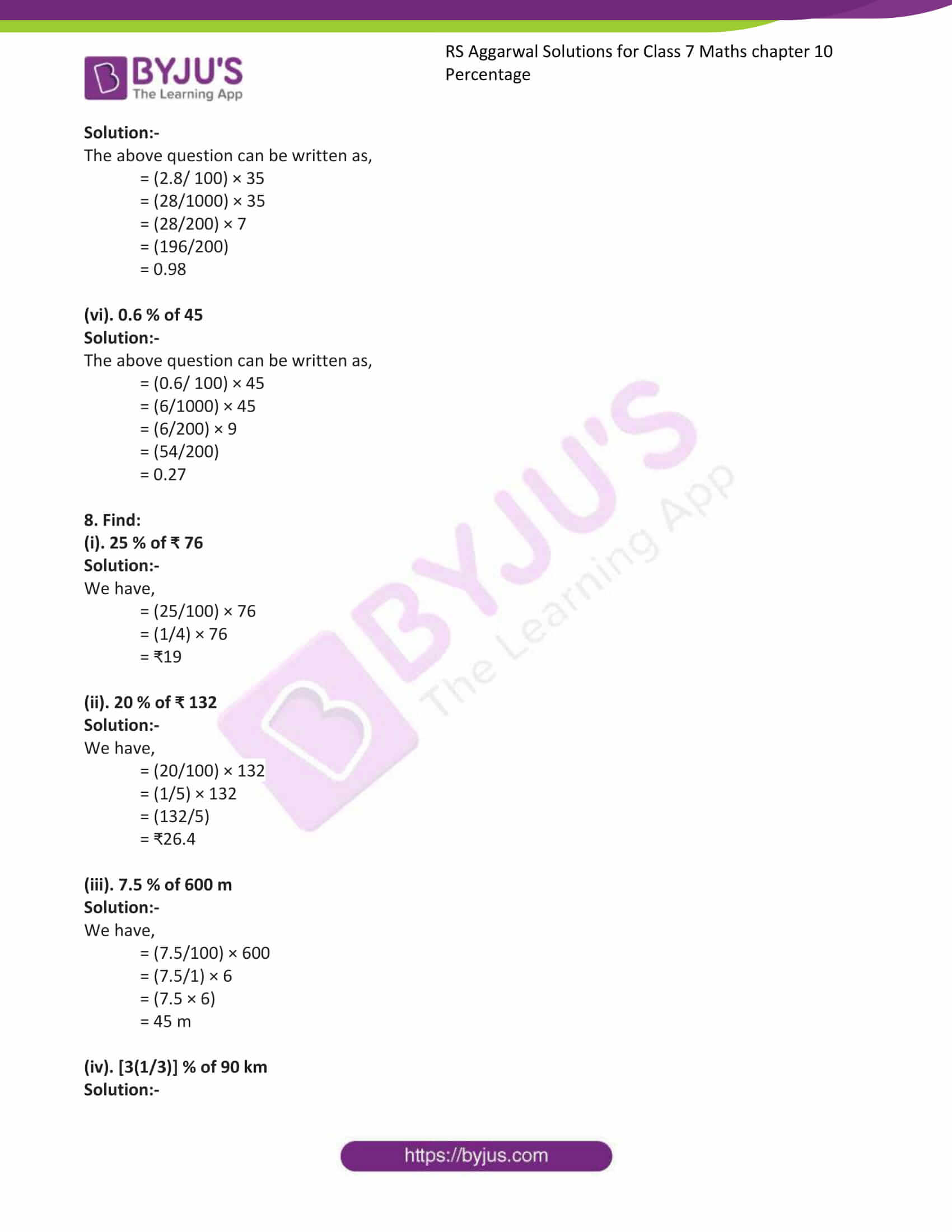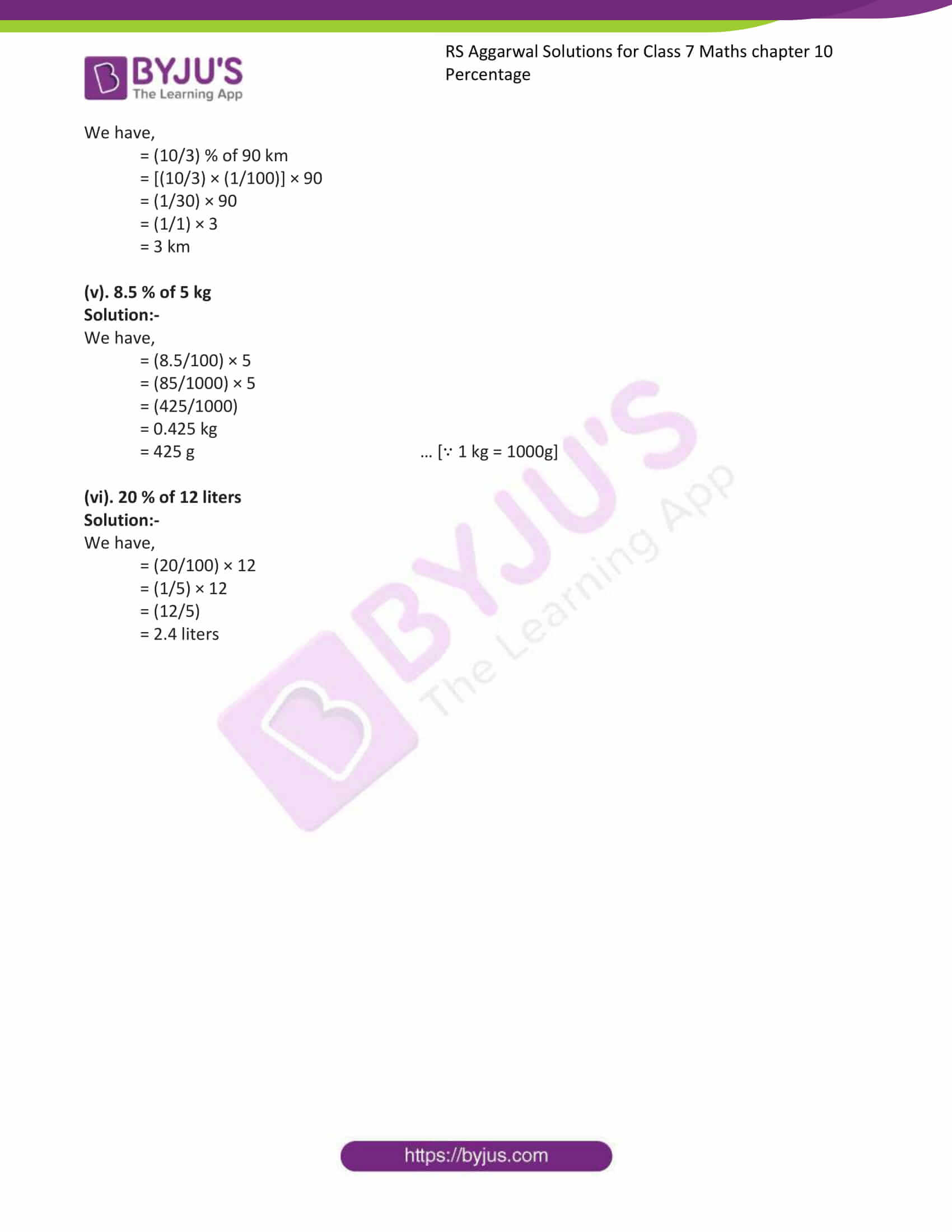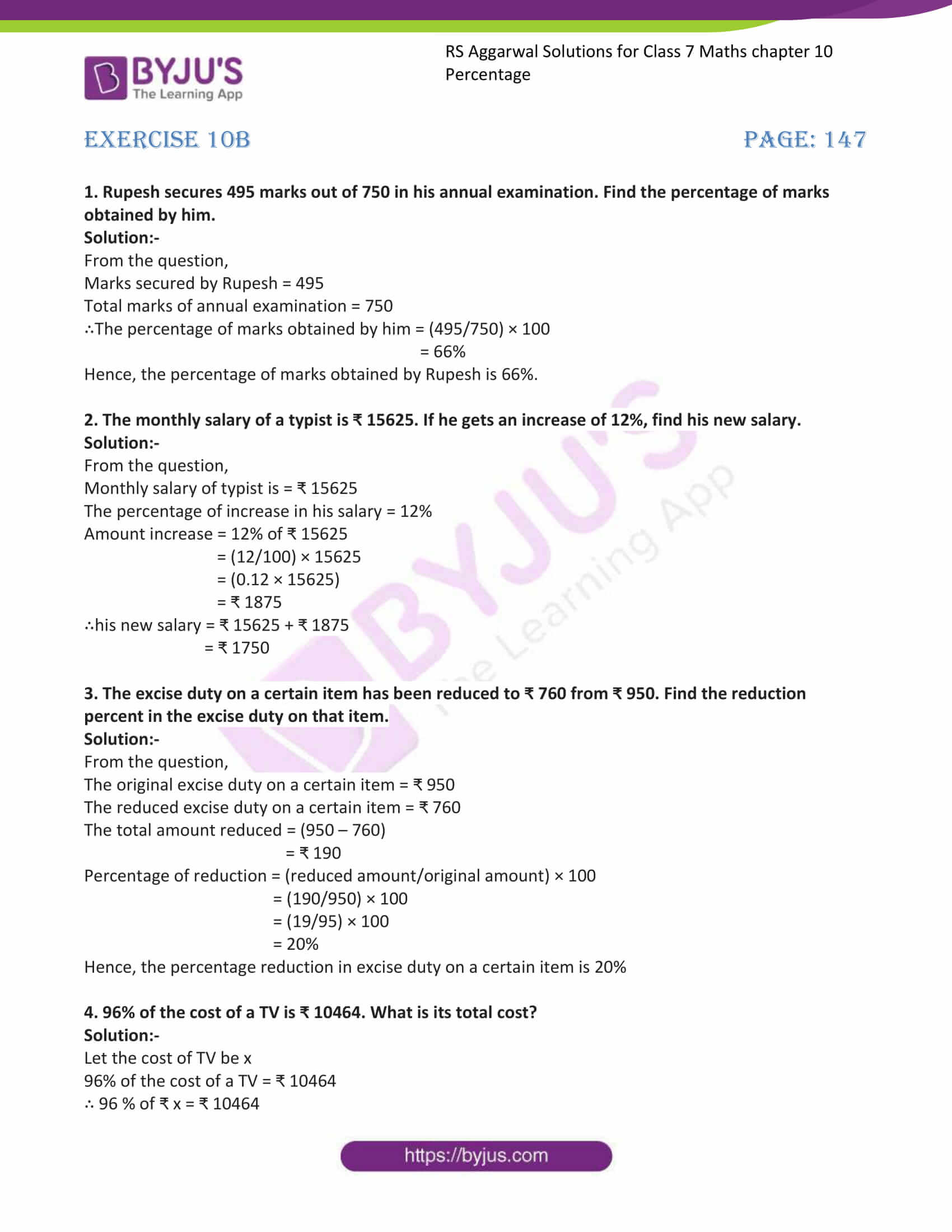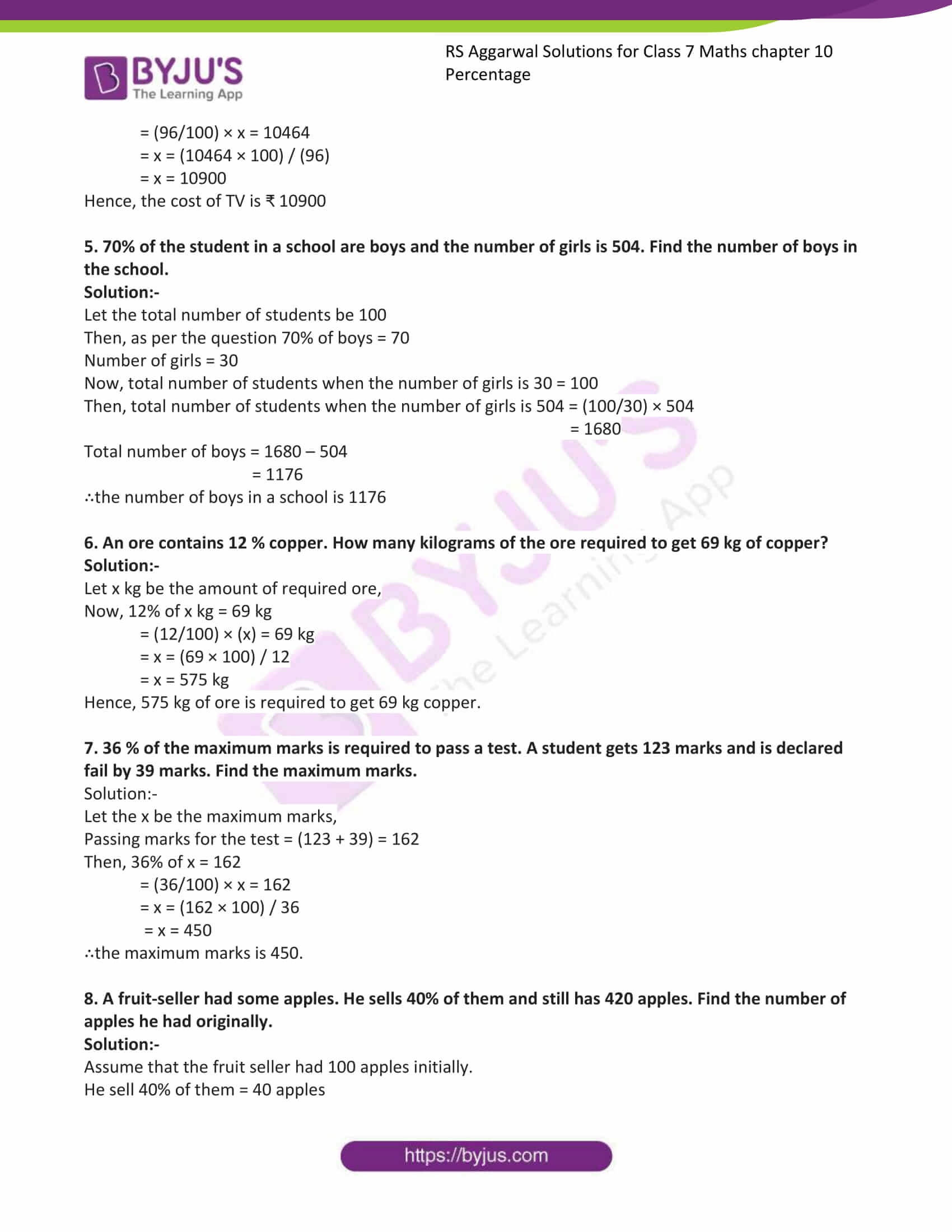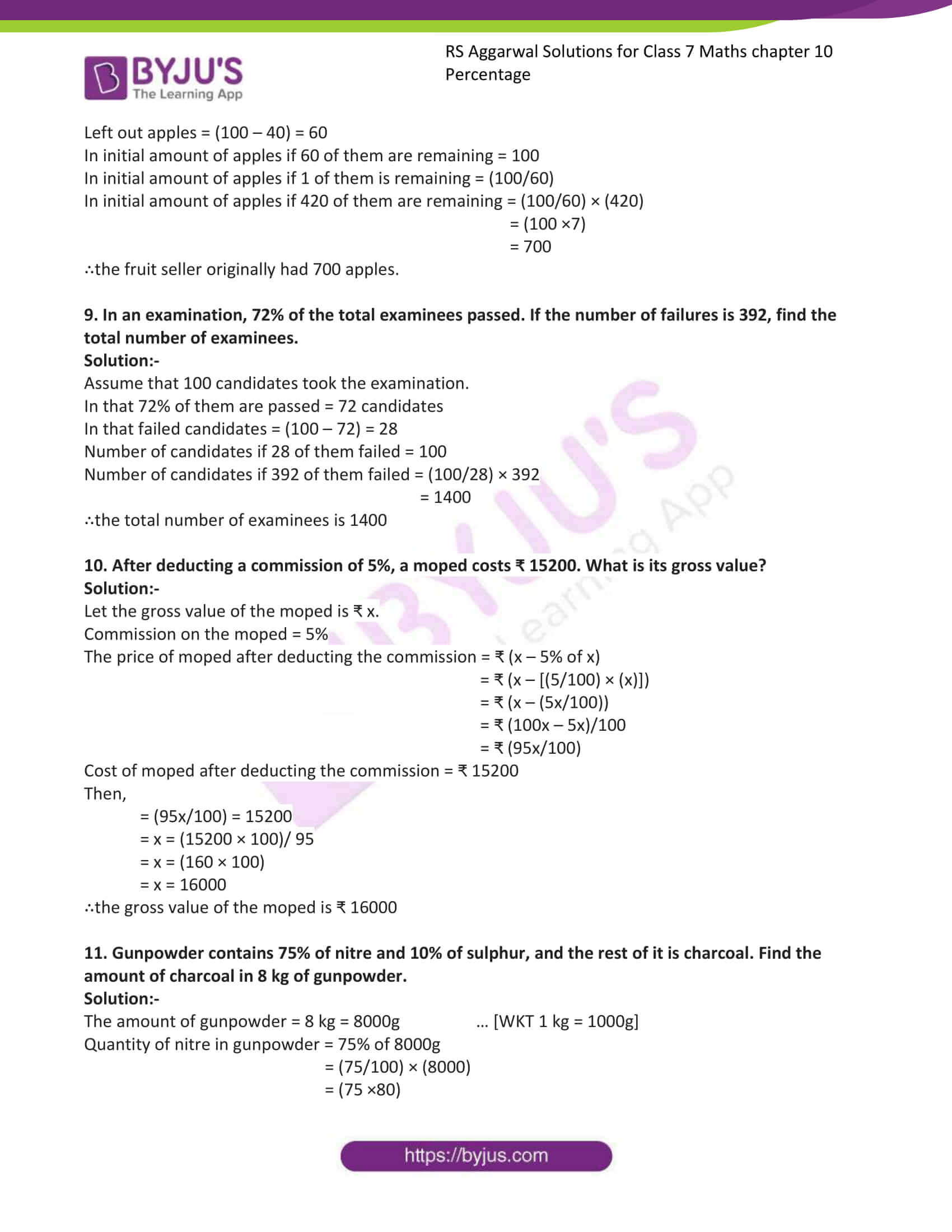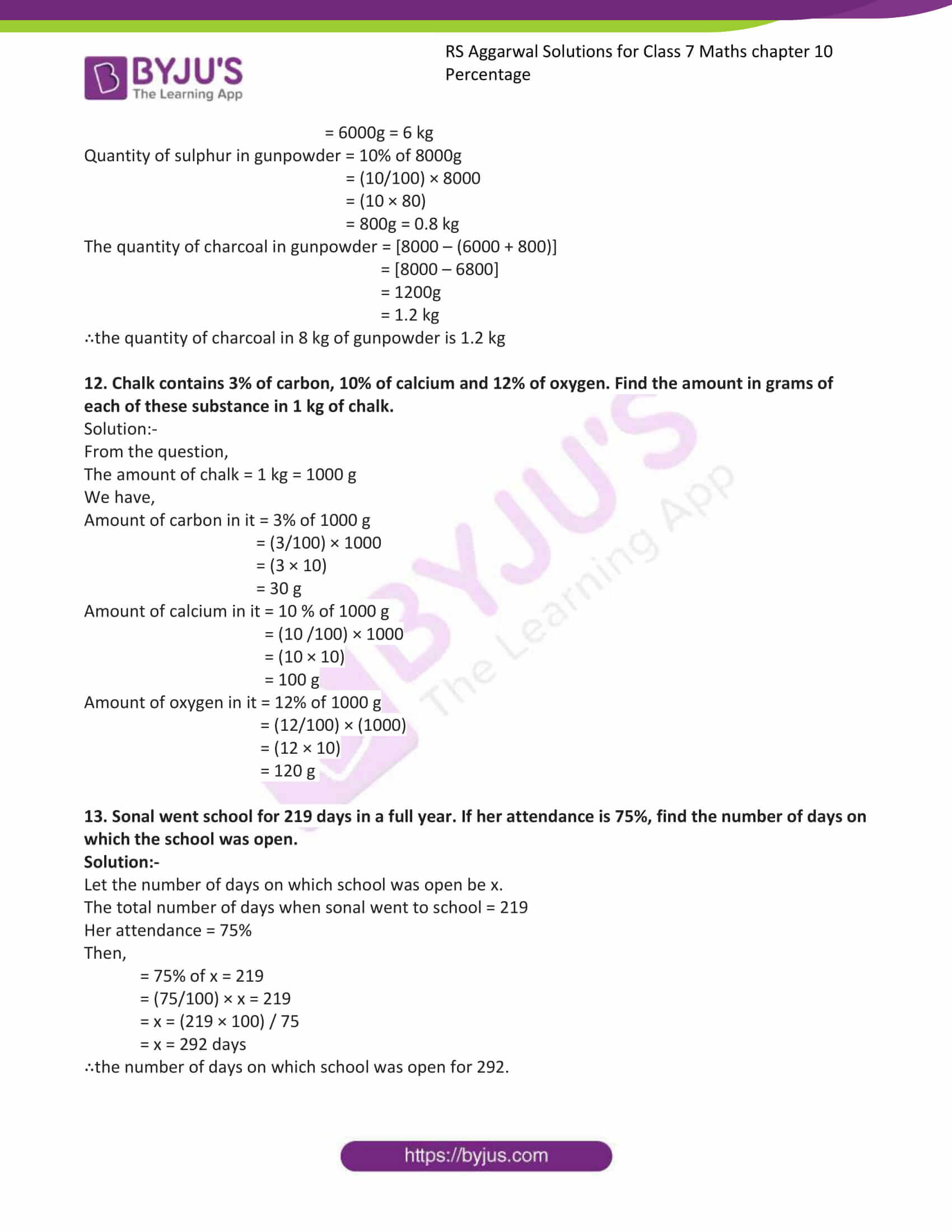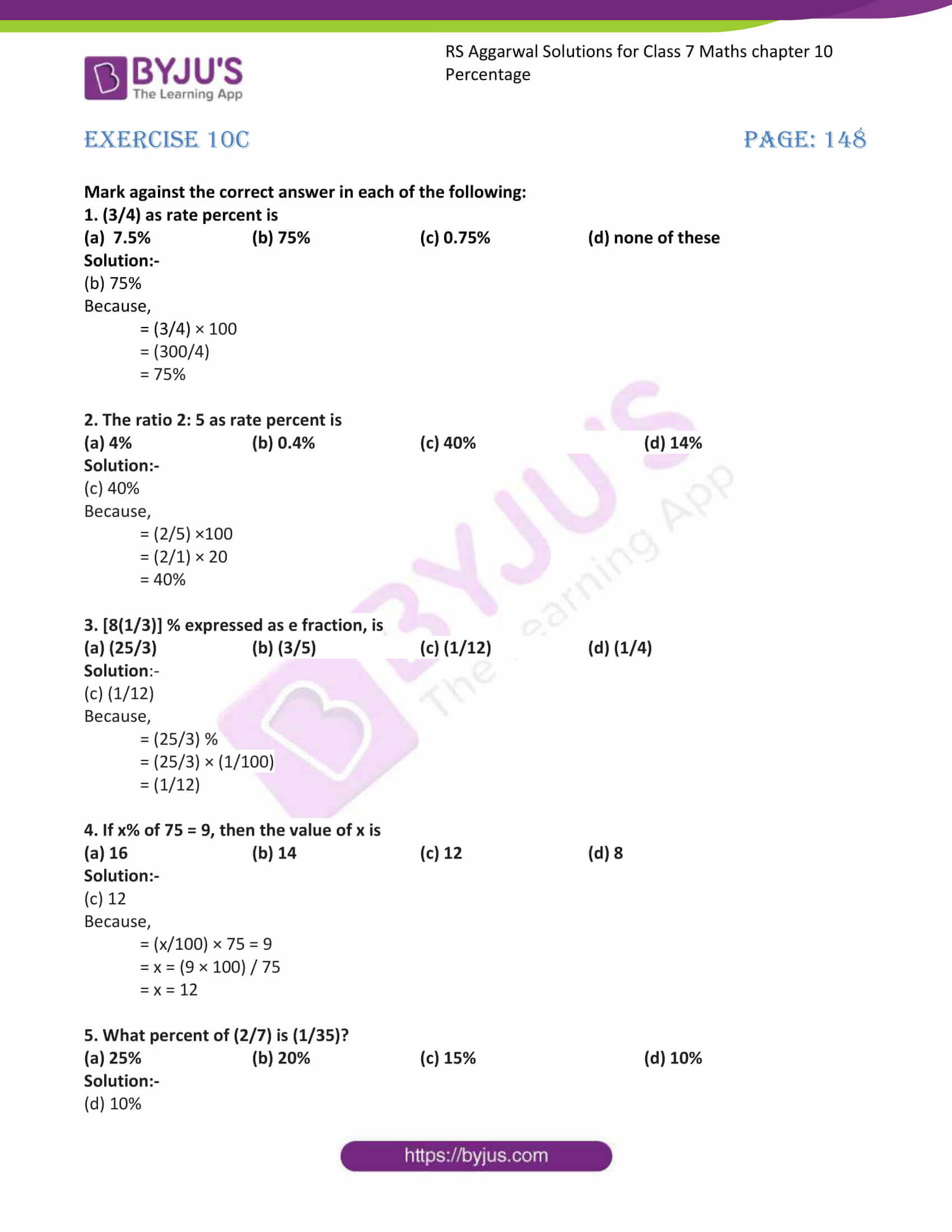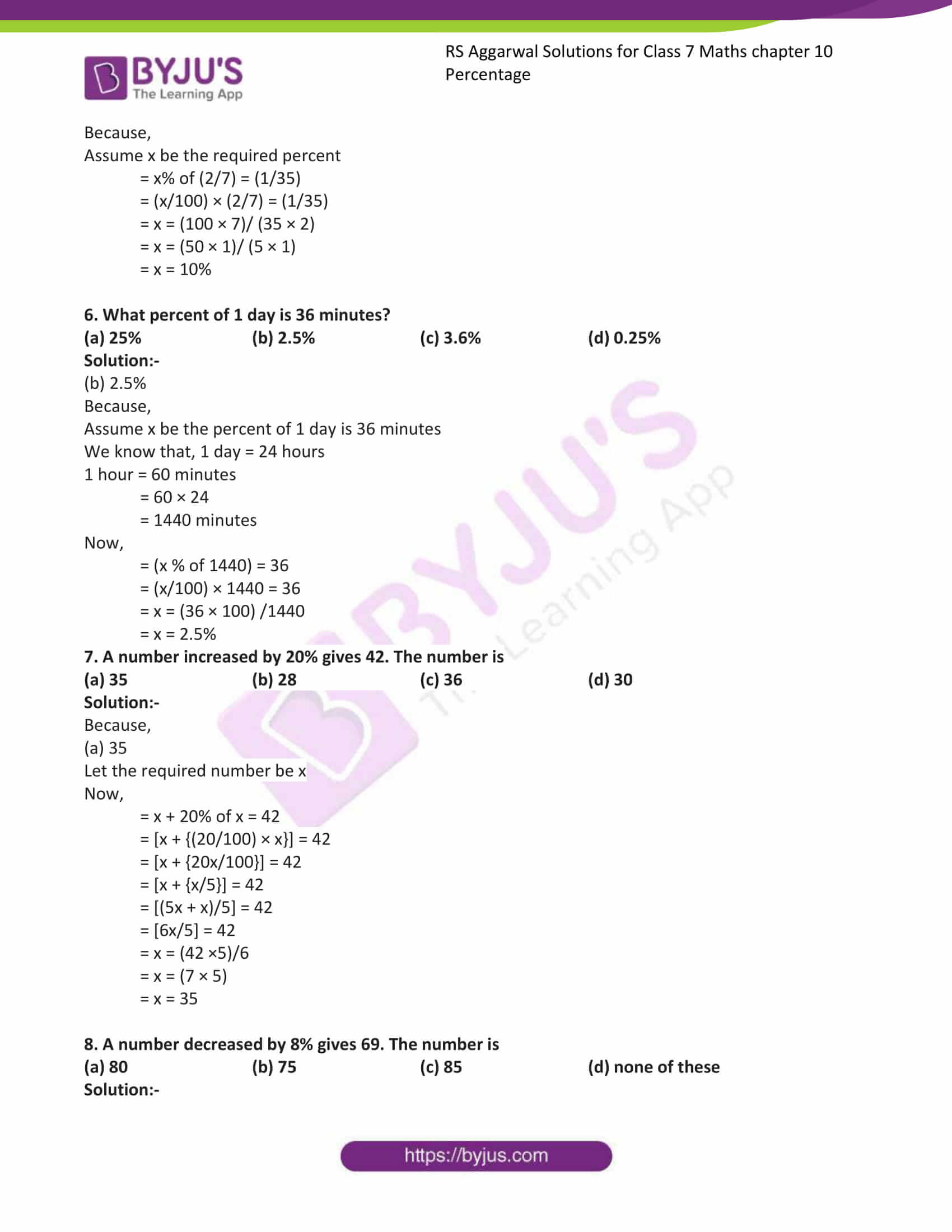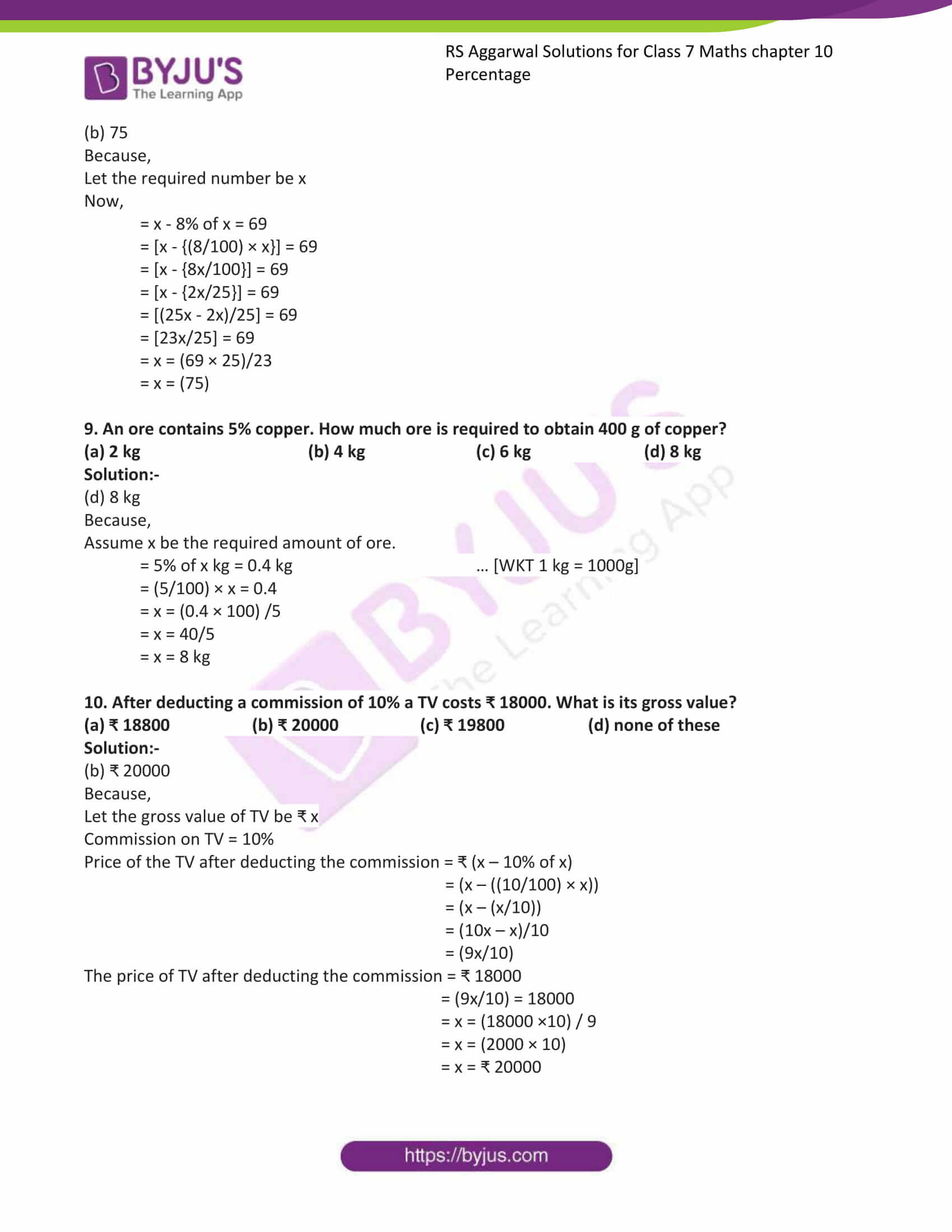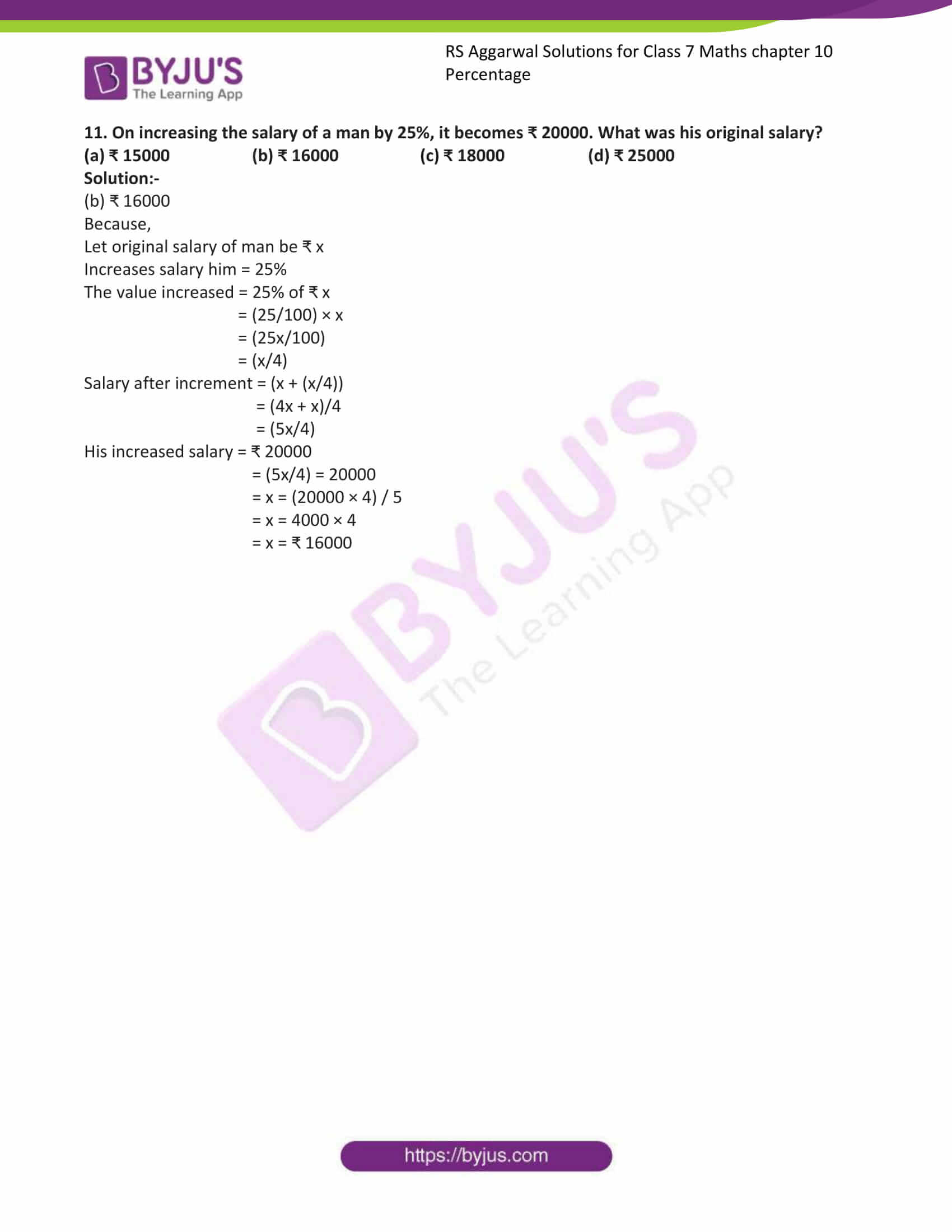## Exercise 10A

1. Convert each of the following fractions into a percentage:

(i). (47/100)

Solution:-

In order to convert a fraction into a percentage multiply the fraction by 100 and put the percent sign %.

= (47/100) × 100

= 47%

(ii). (9/20)

Solution:-

In order to convert a fraction into a percentage multiply the fraction by 100 and put the percent sign %.

= (9/20) × 100

= 9 × 5

= 45%

(iii). (3/8)

Solution:-

In order to convert a fraction into a percentage multiply the fraction by 100 and put the percent sign %.

= (3/8) × 100

= (3/2) × 25

= (75/2)

= 37.5%

(iv). (8/125)

Solution:-

In order to convert a fraction into a percentage multiply the fraction by 100 and put the percent sign %.

= (8/125) × 100

= (8/5) × 4

= (32/5)

= 37(1/2) %

(v). (19/500)

Solution:-

In order to convert a fraction into a percentage multiply the fraction by 100 and put the percent sign %.

= (19/500) × 100

= (19/5)

= 3.8%

(vi). (4/15)

Solution:-

In order to convert a fraction into a percentage multiply the fraction by 100 and put the percent sign %.

= (4/15) × 100

= (4/3) × 20

= 26(2/3) %

(vii). (2/3)

Solution:-

In order to convert a fraction into a percentage multiply the fraction by 100 and put the percent sign %.

= (2/3) × 100

= (200/3)

= 66(2/3) %

(viii). [1(3/5)]

Solution:-

In order to convert a fraction into a percentage multiply the fraction by 100 and put the percent sign %.

= [1(3/5)] × 100

= (8/5) × 100

= (8 × 20)

= 160 %

2. Convert each of the following into a fraction:

(i). 32%

Solution:-

To convert a percentage into a fraction, we divide it by 100 and remove the sign %.

We have,

= (32/100)

= 8/25

(ii). [6(1/4)] %

Solution:-

To convert a percentage into a fraction, we divide it by 100 and remove the sign %.

We have,

= (25/4)

= (25/4)/100

= (25/4) × (1/100)

= (1/4) × (1/4)

= 1/16

(iii). [26(2/3)] %

Solution:-

To convert a percentage into a fraction, we divide it by 100 and remove the sign %.

We have,

= (80/3)

= (80/3)/100

= (80/3) × (1/100)

= (4/3) × (1/5)

= 4/15

(iv). 120 %

Solution:-

To convert a percentage into a fraction, we divide it by 100 and remove the sign %.

We have,

= (120)/100

= (6/5)

= [1(1/5)]

(v). 6.25 %

Solution:-

To convert a percentage into a fraction, we divide it by 100 and remove the sign %.

We have,

= (6.25)/100

= (625/10000)

= (25/400)

= (1/16)

(vi). 0.8 %

Solution:-

To convert a percentage into a fraction, we divide it by 100 and remove the sign %.

We have,

= (0.8)/100

= (8/1000)

= (1/125)

(vii). 0.06 %

Solution:-

To convert a percentage into a fraction, we divide it by 100 and remove the sign %.

We have,

= (0.06)/100

= (6/10000)

= (3/5000)

(viii). 22.75 %

Solution:-

To convert a percentage into a fraction, we divide it by 100 and remove the sign %.

We have,

= (22.75)/100

= (2275/10000)

= (91/400)

3. Express each of the following as a ratio:

(i). 43%

Solution:-

Percentage can be expressed as a ratio with its second term 100 and first term equal to the given percentage.

We have,

= (43/100)

= 43: 100

(ii). 36%

Solution:-

Percentage can be expressed as a ratio with its second term 100 and first term equal to the given percentage.

We have,

= (36/100)

= 36: 100

(iii). 7.5%

Solution:-

Percentage can be expressed as a ratio with its second term 100 and first term equal to the given percentage.

We have,

= (7.5/100)

= (75/1000)

= 3/40

= 3: 40

(iv). 125%

Solution:-

Percentage can be expressed as a ratio with its second term 100 and first term equal to the given percentage.

We have,

= (125/100)

= 5/4

= 5: 4

4. Convert each of the following ratios into a percentage:

(i). 37: 100

Solution:-

First convert the given ratio into fraction, and then multiply the fraction by 100 and put the percent sign %.

= (37/100)

= (37/100) × 100

= 37 %

(ii). 16: 25

Solution:-

First convert the given ratio into fraction, and then multiply the fraction by 100 and put the percent sign %.

= (16/25)

= (16/25) × 100

= (16 × 4)

= 64 %

(iii). 3: 5

Solution:-

First convert the given ratio into fraction, and then multiply the fraction by 100 and put the percent sign %.

= (3/5)

= (3/5) × 100

= (3 × 20)

= 60 %

(iv). 5: 4

Solution:-

First convert the given ratio into fraction, and then multiply the fraction by 100 and put the percent sign %.

= (5/4)

= (5/4) × 100

= (5× 25)

= 125 %

5. Convert the each of the following into decimal form:

(i). 45%

Solution:-

First convert the given percentage into fraction and then put the fraction into decimal form.

= (45/100)

= 0.45

(ii). 127%

Solution:-

First convert the given percentage into fraction and then put the fraction into decimal form.

= (127/100)

= 1.27

(iii). 3.6%

Solution:-

First convert the given percentage into fraction and then put the fraction into decimal form.

= (3.6/100)

= (36/1000)

= 0.036

(iv). 0.23%

Solution:-

First convert the given percentage into fraction and then put the fraction into decimal form.

= (0.23/100)

= (23/10000)

= 0.0023

6. Convert each of the following decimals into a percentage:

(i). 0.6

Solution:-

Multiply the given decimal by 100 and put the percent sign %.

We have,

= (0.6 × 100)

= 60%

(ii). 0.42

Solution:-

Multiply the given decimal by 100 and put the percent sign %.

We have,

= (0.42 × 100)

= 42%

(iii). 0.07

Solution:-

Multiply the given decimal by 100 and put the percent sign %.

We have,

= (0.07 × 100)

= 7%

(iv). 0.005

Solution:-

Multiply the given decimal by 100 and put the percent sign %.

We have,

= (0.005 × 100)

= 0.5%

7. Find:

(i). 32% of 425

Solution:-

= (32/100) × 425

= (32/4) × 17

= (8 × 17)

= 136

(ii). [16(2/3)] % of 16

Solution:-

The above question can be written as,

= (50/3) % of 16

= [(50/3) / (100)] × 16

= [(50/3) × (1/100)] × 16

= (1/6) × 16

= (8/3)

= [2(2/3)]

(iii). 6.5 % of 400

Solution:-

The above question can be written as,

= (6.5 / 100) × 400

= (65/1000) × 400

= (65/10) × 4

= (260/10)

= 26

(iv). 136 % of 70

Solution:-

The above question can be written as,

= (136 / 100) × 70

= (136/10) × 7

= (952/10)

= 95.2

(v). 2.8 % of 35

Solution:-

The above question can be written as,

= (2.8/ 100) × 35

= (28/1000) × 35

= (28/200) × 7

= (196/200)

= 0.98

(vi). 0.6 % of 45

Solution:-

The above question can be written as,

= (0.6/ 100) × 45

= (6/1000) × 45

= (6/200) × 9

= (54/200)

= 0.27

8. Find:

(i). 25 % of ₹ 76

Solution:-

We have,

= (25/100) × 76

= (1/4) × 76

= ₹19

(ii). 20 % of ₹ 132

Solution:-

We have,

= (20/100) × 132

= (1/5) × 132

= (132/5)

= ₹26.4

(iii). 7.5 % of 600 m

Solution:-

We have,

= (7.5/100) × 600

= (7.5/1) × 6

= (7.5 × 6)

= 45 m

(iv). [3(1/3)] % of 90 km

Solution:-

We have,

= (10/3) % of 90 km

= [(10/3) × (1/100)] × 90

= (1/30) × 90

= (1/1) × 3

= 3 km

(v). 8.5 % of 5 kg

Solution:-

We have,

= (8.5/100) × 5

= (85/1000) × 5

= (425/1000)

= 0.425 kg

= 425 g … [∵ 1 kg = 1000g]

(vi). 20 % of 12 liters

Solution:-

We have,

= (20/100) × 12

= (1/5) × 12

= (12/5)

= 2.4 liters

## Exercise 10B

1. Rupesh secures 495 marks out of 750 in his annual examination. Find the percentage of marks obtained by him.

Solution:-

From the question,

Marks secured by Rupesh = 495

Total marks of annual examination = 750

∴The percentage of marks obtained by him = (495/750) × 100

= 66%

Hence, the percentage of marks obtained by Rupesh is 66%.

2. The monthly salary of a typist is ₹ 15625. If he gets an increase of 12%, find his new salary.

Solution:-

From the question,

Monthly salary of typist is = ₹ 15625

The percentage of increase in his salary = 12%

Amount increase = 12% of ₹ 15625

= (12/100) × 15625

= (0.12 × 15625)

= ₹ 1875

∴his new salary = ₹ 15625 + ₹ 1875

= ₹ 1750

3. The excise duty on a certain item has been reduced to ₹ 760 from ₹ 950. Find the reduction percent in the excise duty on that item.

Solution:-

From the question,

The original excise duty on a certain item = ₹ 950

The reduced excise duty on a certain item = ₹ 760

The total amount reduced = (950 – 760)

= ₹ 190

Percentage of reduction = (reduced amount/original amount) × 100

= (190/950) × 100

= (19/95) × 100

= 20%

Hence, the percentage reduction in excise duty on a certain item is 20%

4. 96% of the cost of a TV is ₹ 10464. What is its total cost?

Solution:-

Let the cost of TV be x

96% of the cost of a TV = ₹ 10464

∴ 96 % of ₹ x = ₹ 10464

= (96/100) × x = 10464

= x = (10464 × 100) / (96)

= x = 10900

Hence, the cost of TV is ₹ 10900

5. 70% of the student in a school are boys and the number of girls is 504. Find the number of boys in the school.

Solution:-

Let the total number of students be 100

Then, as per the question 70% of boys = 70

Number of girls = 30

Now, total number of students when the number of girls is 30 = 100

Then, total number of students when the number of girls is 504 = (100/30) × 504

= 1680

Total number of boys = 1680 – 504

= 1176

∴the number of boys in a school is 1176

6. An ore contains 12 % copper. How many kilograms of the ore required to get 69 kg of copper?

Solution:-

Let x kg be the amount of required ore,

Now, 12% of x kg = 69 kg

= (12/100) × (x) = 69 kg

= x = (69 × 100) / 12

= x = 575 kg

Hence, 575 kg of ore is required to get 69 kg copper.

7. 36 % of the maximum marks is required to pass a test. A student gets 123 marks and is declared fail by 39 marks. Find the maximum marks.

Solution:-

Let the x be the maximum marks,

Passing marks for the test = (123 + 39) = 162

Then, 36% of x = 162

= (36/100) × x = 162

= x = (162 × 100) / 36

= x = 450

∴the maximum marks is 450.

8. A fruit-seller had some apples. He sells 40% of them and still has 420 apples. Find the number of apples he had originally.

Solution:-

Assume that the fruit seller had 100 apples initially.

He sell 40% of them = 40 apples

Left out apples = (100 – 40) = 60

In initial amount of apples if 60 of them are remaining = 100

In initial amount of apples if 1 of them is remaining = (100/60)

In initial amount of apples if 420 of them are remaining = (100/60) × (420)

= (100 ×7)

= 700

∴the fruit seller originally had 700 apples.

9. In an examination, 72% of the total examinees passed. If the number of failures is 392, find the total number of examinees.

Solution:-

Assume that 100 candidates took the examination.

In that 72% of them are passed = 72 candidates

In that failed candidates = (100 – 72) = 28

Number of candidates if 28 of them failed = 100

Number of candidates if 392 of them failed = (100/28) × 392

= 1400

∴the total number of examinees is 1400

10. After deducting a commission of 5%, a moped costs ₹ 15200. What is its gross value?

Solution:-

Let the gross value of the moped is ₹ x.

Commission on the moped = 5%

The price of moped after deducting the commission = ₹ (x – 5% of x)

= ₹ (x – [(5/100) × (x)])

= ₹ (x – (5x/100))

= ₹ (100x – 5x)/100

= ₹ (95x/100)

Cost of moped after deducting the commission = ₹ 15200

Then,

= (95x/100) = 15200

= x = (15200 × 100)/ 95

= x = (160 × 100)

= x = 16000

∴the gross value of the moped is ₹ 16000

11. Gunpowder contains 75% of nitre and 10% of sulphur, and the rest of it is charcoal. Find the amount of charcoal in 8 kg of gunpowder.

Solution:-

The amount of gunpowder = 8 kg = 8000g … [WKT 1 kg = 1000g]

Quantity of nitre in gunpowder = 75% of 8000g

= (75/100) × (8000)

= (75 ×80)

= 6000g = 6 kg

Quantity of sulphur in gunpowder = 10% of 8000g

= (10/100) × 8000

= (10 × 80)

= 800g = 0.8 kg

The quantity of charcoal in gunpowder = [8000 – (6000 + 800)]

= [8000 – 6800]

= 1200g

= 1.2 kg

∴the quantity of charcoal in 8 kg of gunpowder is 1.2 kg

12. Chalk contains 3% of carbon, 10% of calcium and 12% of oxygen. Find the amount in grams of each of these substance in 1 kg of chalk.

Solution:-

From the question,

The amount of chalk = 1 kg = 1000 g

We have,

Amount of carbon in it = 3% of 1000 g

= (3/100) × 1000

= (3 × 10)

= 30 g

Amount of calcium in it = 10 % of 1000 g

= (10 /100) × 1000

= (10 × 10)

= 100 g

Amount of oxygen in it = 12% of 1000 g

= (12/100) × (1000)

= (12 × 10)

= 120 g

13. Sonal went school for 219 days in a full year. If her attendance is 75%, find the number of days on which the school was open.

Solution:-

Let the number of days on which school was open be x.

The total number of days when sonal went to school = 219

Her attendance = 75%

Then,

= 75% of x = 219

= (75/100) × x = 219

= x = (219 × 100) / 75

= x = 292 days

∴the number of days on which school was open for 292.

## Exercise 10C

Mark against the correct answer in each of the following:

1. (3/4) as rate percent is

(a) 7.5% (b) 75% (c) 0.75% (d) none of these

Solution:-

(b) 75%

Because,

= (3/4) × 100

= (300/4)

= 75%

2. The ratio 2: 5 as rate percent is

(a) 4% (b) 0.4% (c) 40% (d) 14%

Solution:-

(c) 40%

Because,

= (2/5) ×100

= (2/1) × 20

= 40%

3. [8(1/3)] % expressed as e fraction, is

(a) (25/3) (b) (3/5) (c) (1/12) (d) (1/4)

Solution:-

(c) (1/12)

Because,

= (25/3) %

= (25/3) × (1/100)

= (1/12)

4. If x% of 75 = 9, then the value of x is

(a) 16 (b) 14 (c) 12 (d) 8

Solution:-

(c) 12

Because,

= (x/100) × 75 = 9

= x = (9 × 100) / 75

= x = 12

5. What percent of (2/7) is (1/35)?

(a) 25% (b) 20% (c) 15% (d) 10%

Solution:-

(d) 10%

Because,

Assume x be the required percent

= x% of (2/7) = (1/35)

= (x/100) × (2/7) = (1/35)

= x = (100 × 7)/ (35 × 2)

= x = (50 × 1)/ (5 × 1)

= x = 10%

6. What percent of 1 day is 36 minutes?

(a) 25% (b) 2.5% (c) 3.6% (d) 0.25%

Solution:-

(b) 2.5%

Because,

Assume x be the percent of 1 day is 36 minutes

We know that, 1 day = 24 hours

1 hour = 60 minutes

= 60 × 24

= 1440 minutes

Now,

= (x % of 1440) = 36

= (x/100) × 1440 = 36

= x = (36 × 100) /1440

= x = 2.5%

7. A number increased by 20% gives 42. The number is

(a) 35 (b) 28 (c) 36 (d) 30

Solution:-

Because,

(a) 35

Let the required number be x

Now,

= x + 20% of x = 42

= [x + {(20/100) × x}] = 42

= [x + {20x/100}] = 42

= [x + {x/5}] = 42

= [(5x + x)/5] = 42

= [6x/5] = 42

= x = (42 ×5)/6

= x = (7 × 5)

= x = 35

8. A number decreased by 8% gives 69. The number is

(a) 80 (b) 75 (c) 85 (d) none of these

Solution:-

(b) 75

Because,

Let the required number be x

Now,

= x – 8% of x = 69

= [x – {(8/100) × x}] = 69

= [x – {8x/100}] = 69

= [x – {2x/25}] = 69

= [(25x – 2x)/25] = 69

= [23x/25] = 69

= x = (69 × 25)/23

= x = (75)

9. An ore contains 5% copper. How much ore is required to obtain 400 g of copper?

(a) 2 kg (b) 4 kg (c) 6 kg (d) 8 kg

Solution:-

(d) 8 kg

Because,

Assume x be the required amount of ore.

= 5% of x kg = 0.4 kg … [WKT 1 kg = 1000g]

= (5/100) × x = 0.4

= x = (0.4 × 100) /5

= x = 40/5

= x = 8 kg

10. After deducting a commission of 10% a TV costs ₹ 18000. What is its gross value?

(a) ₹ 18800 (b) ₹ 20000 (c) ₹ 19800 (d) none of these

Solution:-

(b) ₹ 20000

Because,

Let the gross value of TV be ₹ x

Commission on TV = 10%

Price of the TV after deducting the commission = ₹ (x – 10% of x)

= (x – ((10/100) × x))

= (x – (x/10))

= (10x – x)/10

= (9x/10)

The price of TV after deducting the commission = ₹ 18000

= (9x/10) = 18000

= x = (18000 ×10) / 9

= x = (2000 × 10)

= x = ₹ 20000

11. On increasing the salary of a man by 25%, it becomes ₹ 20000. What was his original salary?

(a) ₹ 15000 (b) ₹ 16000 (c) ₹ 18000 (d) ₹ 25000

Solution:-

(b) ₹ 16000

Because,

Let original salary of man be ₹ x

Increases salary him = 25%

The value increased = 25% of ₹ x

= (25/100) × x

= (25x/100)

= (x/4)

Salary after increment = (x + (x/4))

= (4x + x)/4

= (5x/4)

His increased salary = ₹ 20000

= (5x/4) = 20000

= x = (20000 × 4) / 5

= x = 4000 × 4

= x = ₹ 16000

## RS Aggarwal Solutions for Class 7 Maths Chapter 10 – Percentage

Chapter 10 – Percentage contains 3 exercises and the RS Aggarwal Solutions available on this page provide solutions to the questions present in the exercises. Now, let us have a look at some of the concepts discussed in this chapter.

• To Convert a Fraction into a Percentage
• To Convert a Percentage into a Fraction
• Percentage as a Ratio
• To Convert a Given Ratio into a Percentage
• To Convert a Percentage in Decimal Form
• To convert a Given Decimal into a Percentage
• Word Problems On Percentage

### Chapter Brief of RS Aggarwal Solutions for Class 7 Maths Chapter 10 – Percentage

RS Aggarwal Solutions for Class 7 Maths Chapter 10 – Percentage. This chapter covers the concept of percentage and study percentage as a fraction, as a ratio and also learn about the conversion of a percent into fraction, ratio or decimal and vice-versa. The solutions are solved in such a way that students will understand clearly, and they will never get confused.